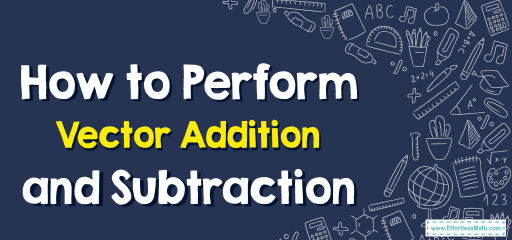# How to Perform Vector Addition and Subtraction

Vector addition and subtraction are fundamental operations in vector algebra used to combine or differentiate vectors. Let's break down the concepts.## Step-by-step Guide to Perform Vector Addition and Subtraction

Here is a step-by-step guide to perform vector addition and subtraction:

### Prelude: Grasping the Essence of Vectors

1. Vector Definition: A vector is a mathematical entity with both magnitude and direction. They are often depicted as arrows where the length represents the magnitude and the arrow’s direction denotes its direction.
2. Vector Components: In a Cartesian coordinate system, a vector in two dimensions can be represented as $$v=(v_x​, v_y​)$$, where $$v_x$$ and $$v_y$$​ are the $$x$$ and $$y$$ components respectively.

### Chapter I: Vector Addition – The Confluence of Magnitudes and Directions

• $$v+w=(v_x​ + w_x​, v_y + w_y)$$
2. Graphical Method:
• Initiation: Draw the first vector starting from the origin.
• Continuation: From the head of the first vector, draw the second vector.
• Conclusion: The resultant vector (sum) starts from the origin and ends at the head of the second vector.
3. Summarizing the Process: The process is akin to walking a certain distance in one direction (first vector) and then continuing from there in another direction (second vector).

### Chapter II: Vector Subtraction – The Divergence of Pathways

1. Component-wise Subtraction: Subtracting vectors is analogous to addition but involves taking away magnitudes.
• $$v+w=(v_x​ – w_x​, v_y – w_y)$$
2. Graphical Method:
• Initiation: Begin by drawing the first vector, $$v$$, starting from the origin.
• Introduction of the Negative Vector: Visualize or sketch the negative of the second vector, $$−w$$. This is a vector of the same magnitude as $$w$$ but in the opposite direction.
• Continuation: From the head of $$v$$, draw $$−w$$.
• Conclusion: The resultant vector (difference) begins at the origin and ends at the head of $$−w$$.
3. Summarizing the Process: Imagine retracing a portion of a journey. You take your entire journey (first vector) and then move backward by the path denoted by the second vector.

### Postlude: Reflecting on Operations

• Vectors, with their dual nature of magnitude and direction, offer an elegant way to describe physical quantities. When we combine or separate vectors, we’re essentially juggling these two properties in a dance of mathematical harmony.
• Practicing these operations on varied vectors will solidify your understanding and enhance your ability to navigate the world of vector operations.

### Examples:

Example 1:

Given vectors $$a=(5,3)$$ and $$b=(−2,7)$$, find $$a+b$$.

Solution:

$$a+b=(a_x​ + \ b_x​, a_y + \ b_y)=(5+(−2),3+7) =(3,10)$$

So, $$a+b=(3,10)$$.

Example 2:

Given vectors $$p=(7,2)$$ and $$q=(4,5)$$, find $$p−q$$.

Solution:

To subtract vector $$q$$ from vector $$p$$, we subtract the respective components of the two vectors.$$p−q=(p_x​−\ q_x​, p_y​−\ q_y​)=(7−4,2−5)=(3,−3)$$

Thus, $$p−q=(3,−3)$$.

### What people say about "How to Perform Vector Addition and Subtraction - Effortless Math: We Help Students Learn to LOVE Mathematics"?

No one replied yet.

X
30% OFF

Limited time only!

Save Over 30%

SAVE $5 It was$16.99 now it is \$11.99